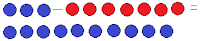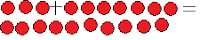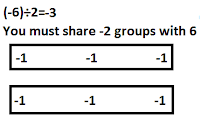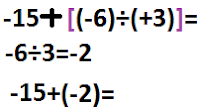## Wednesday, March 9, 2011

### Nikki's Great Big Book of Integers

Chapter 1 Grade 7 Integer Review
REMEMBER!!! When subtracting something that isn't there, use a zero pair.
-3-(-7)=+4-3-7=-103-7=-43+7=10-3+7=-4Great Big Book of Integers Chapter 2
Today in class we were assigned 4 que
stions on multiplying integers. They are:1. (+2)x(+3)=62x3=62.(+2)x(-3)=-6Make +2 groups of -3.

3.(-2)x(+3)=-64.(-2)x(-3)=6
When taking away something that is not there. Use a zero pair.The Great Big Book of Integers Chapter 3

Partitive Division is: When a Problem you know the all the groups and you are trying to find the number of items/objects/things per group. ex. If you have 30 bones and yo
u want to divide them equally with all of the dogs. You have to figure out how many st
icks each dog gets.-6÷(-2)=-3
6÷2=3

Quotative Divisioni
s: When you know the number of items in every group and you are trying to find how many groups there are. If you have 40 dog bones and you have to give 5 bones to every person. You have to find out how many people there are to share the bones.
Multiplive Inverse could of helped by... 6÷(-2)=
6÷(-2)=(-6)÷2=-3Sign Rules for multiplication, division, adding and subtracting

ex.
6÷(-2)=3 There is only one negative sign so the product is negative.
6÷2=3 There are no negative signs in the equation so the answer must be a positive
-6÷(-2)=3 Both of the numbers are negative. Two negatives equal a positive. So the answer is a positive.
-6÷2=-3 The answer must be a negative because there is a odd number of negative signs.

Chapter 4

Order of Operations With Integers

Use BEDMAS to solve the question. But do NOT use E
Reminder:
B-Brackets
E-Exponents(do not use on this question)
D-Division
S-Subtraction

1.First you put square brackets around multiplication, if there is none then division, then addition and finally subtraction.
Square brackets are colored to help you know where they are.2. Put square brackets to the next one in brackets.3. All the steps put into one. And the answer.Thank you for reading my Great Big Book of Integers.